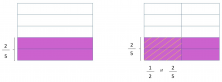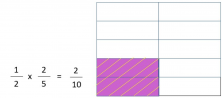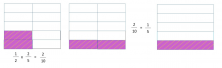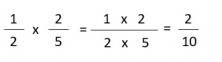Smartick is an online platform for children to master math in only 15 minutes a day

Jun21

# Singapore Math Bar Model for Multiplying Fractions

In today’s post, we’re going to talk about multiplying fractions using the Singapore Math Bar Model. Let’s take a look at an example:

#### Amy drew a rectangle and colored in 2/5 of the parts purple and after, she striped 1/2 of them yellow.We can calculate the answer by observing the described section between the two fractions. That’s to say that 1/2 x 2/5 is equal to 2/10.As we can see in the second picture, the rectangle is divided into 10 parts, 2 of these parts are colored purple and striped yellow; this section is the answer of the multiplication problem, 2/5 by 1/2. Also, we can check the answer by looking at how 2/10 can be reduced to 1/5.The purple 2/10 section has the same amount of parts as the purples 1/5 section. So, in other words, 2/10 and 1/5 are equivalent fractions.I hope that this post helped you understand multiplying fractions with the Singapore Math Bar Model!

Now, onward to some more practice with Elementary Math with Smartick!